Online Tution   »   Practice Paper   »   CBSE Class 12 Maths Additional Practice...

# CBSE Class 12 Maths Additional Practice Questions 2023

## CBSE Class 12 Maths Additional Practice Questions

Check out: CBSE Class 12 Date Sheet 2023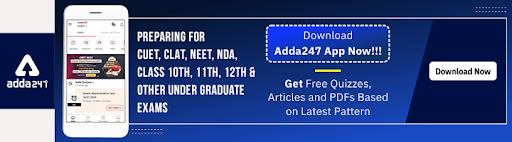## CBSE Class 12 Maths Additional Practice Questions 2023

Time Allowed: 3 Hours
Maximum Marks: 80
General Instructions:
1. This Question paper contains – five sections A, B, C, D and E. Each section is
compulsory. However, there are internal choices in some questions.
2. Section A has 18 MCQs and 02 Assertion-Reason based questions of 1 mark each.
3. Section B has 5 Very Short Answer (VSA)-type questions of 2 marks each.
4. Section C has 6 Short Answer (SA)-type questions of 3 marks each.
5. Section D has 4 Long Answer (LA)-type questions of 5 marks each.
6. Section E has 3 source based/case based/passage based/integrated units of assessment (4 marks each) with sub parts.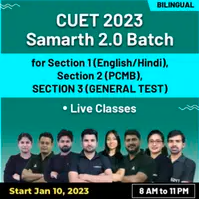## Class 12 Maths Additional Practice Questions 2023

SECTION A
(This section comprises of Multiple-choice questions (MCQ) of 1 mark each.)

Q.1 Shown below is the graph of a function f(x) whose domain is R – (-1,1). Some portion of the graph is hidden behind the star.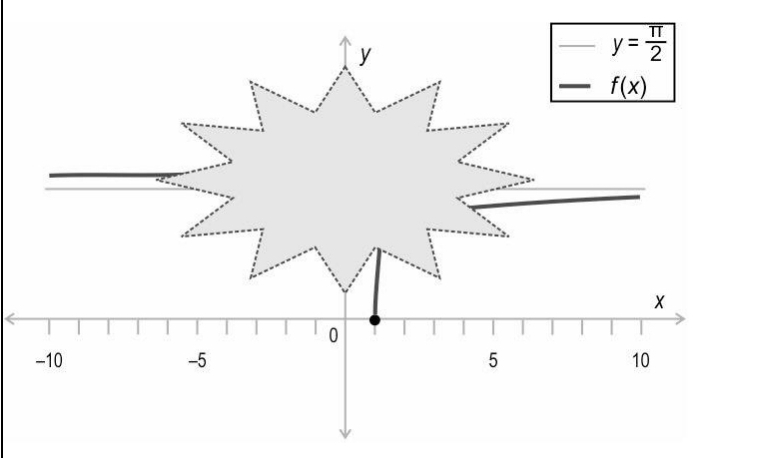Which of the following is f(x)?
A. tan -1x
B. cot-1x
C. sec-1x
D. cosec-1x

Q.2. P and Q are matrices such that both (P + Q) and (PQ) are defined. Which of the following is true about P and Q?
A. P and Q can be any matrices but of the same order.
B. P and Q must be square matrices of the same order.
C. P and Q must be square matrices not necessarily of the same order.
D. Order of P and Q must be of the form m × k and k × n respectively, with no condition on m and n.

Q.3.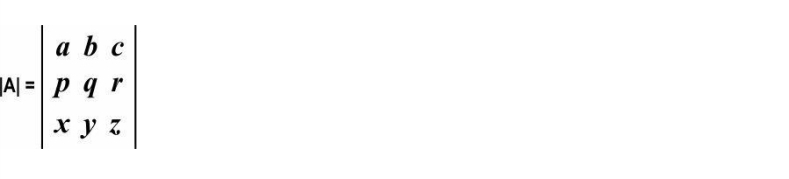Under which of the following conditions will |A| be equal to 0?
i) a – 2p = b – 2q = c – 2r = 0
ii) x = y = z = 0
iii) a : b : c = x : y : z
A. only ii)
B. only i) and ii)
C. only i) and iii)
D. all – i), ii) and iii)

Q.4. If abc = 2, what is the value of the determinant below?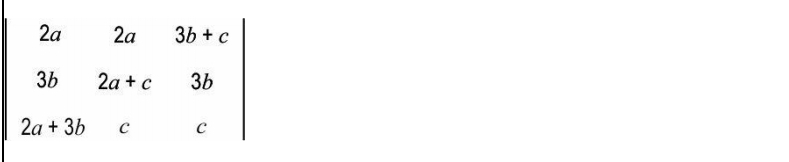A. -48
B. -24
C. 48
D. (cannot be found without the values of a, b and c)

Q.5.For what value of k is the function f continuous at x = 0?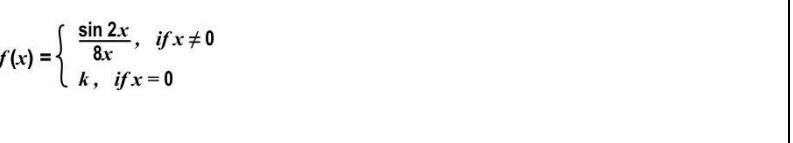A. 4
B. 1
C. ¼
D. 1/8

Q.6 What is the integral of the following expression?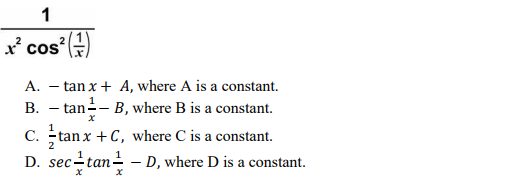Q.7. What is the value of the following integral?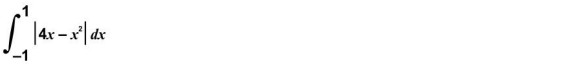A. -4
B. -2
C. 0
D. 4

Q.8. Which of the following is CLOSEST to the area under the parabola given by y = 4x³, bounded by the x-axis, and the lines x = (-1) and x = (-2)?
A. 6 sq units
B. 8 sq units
C. 9 sq units
D. 12 sq units

Q.9 Which of the following differential equation has an order of 2 and a degree of 3?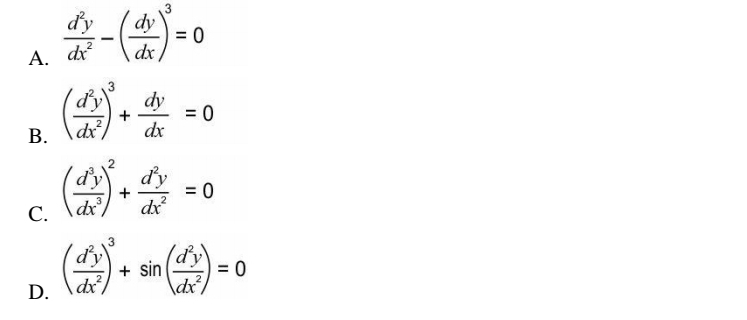Q.10 Following is a differential equation.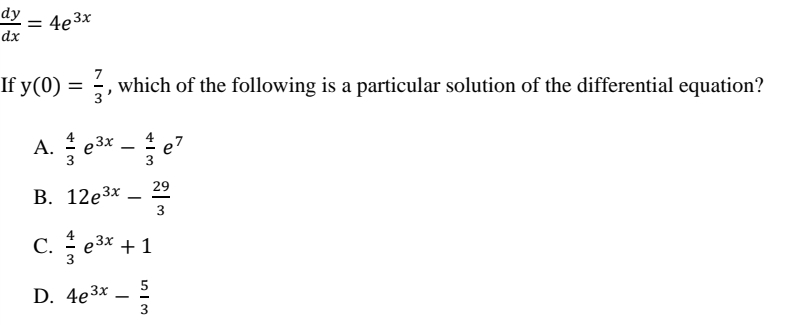Q.11. Shown below is a regular hexagon whose two vertices are joined by a vector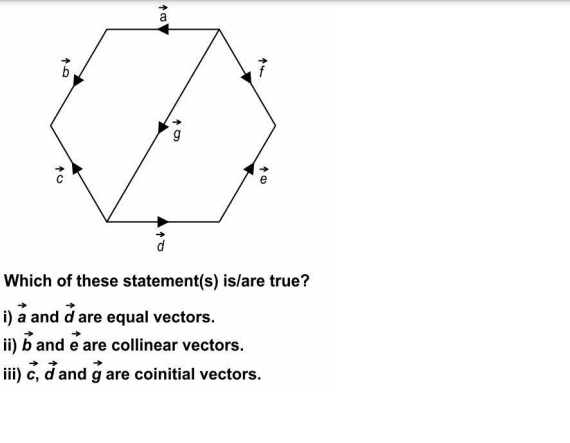A. only ii)
B. only iii)
C. only i) and ii)
D. all – i), ii) and iii)

Q.12.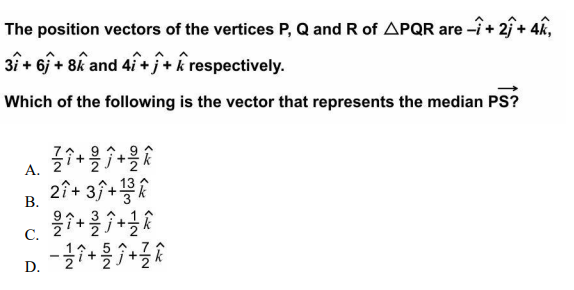Q.13.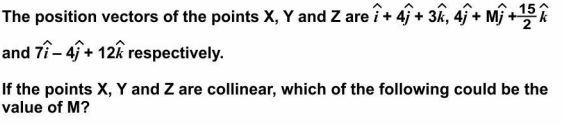A. 8
B. 4
C. 2
D. 0

Q.14.A line makes an angle of 135° with the positive direction of the x-axis, and an angle of 300° with the positive direction of the y-axis. Which of the following could be the angle it makes with the negative direction of the zaxis?
A. 45°
B. 60°
C. (Such a line does not exist.)
D. (A unique angle made with the z-axis cannot be determined.)

Q.15.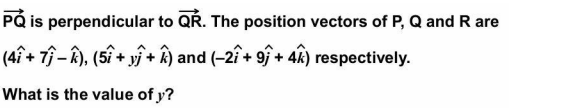Q.16. A linear programming problem (LPP) along with its constraints is given below.
Minimize: 𝑍 = 3x + 2y
Subject to:

x ≤ 4
x ≥ 0, y ≥ 0

Which of the following is true about the above LPP?
A. It has no solution.
B. It has a unique solution.
C. It has two distinct solutions.
D. It has infinitely many solutions.

Q.17. M and N are two events such that P(M|N) = 0.3, P(M)= 0.2 and P(N) = 0.4. Which of the following is the value of P(M ∩ N’)?
A. 0.8
B. 0.12
C. 0.1
D. 0.08

Q.18. The constraints of a linear programming problem along with their graphs is shown below:
x + 2y ≥ 3
x ≥ 10
y ≥ 0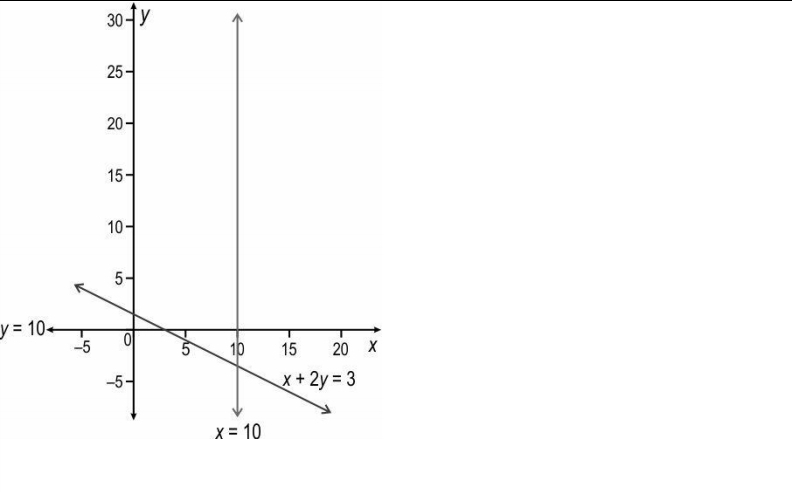Which of the following objective functions has an optimal solution with respect to the
above set of constraints?
A. Minimise Z = x + y
B. Minimise Z = 0.5x + y
C. Maximise Z = x + y
D. Maximise Z = 2x + y

Q.19. Shown below is the graph of function: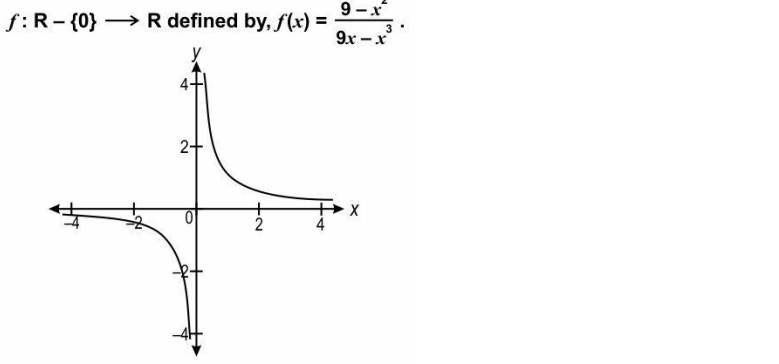Based on the above function, two statements are given below – one labelled Assertion (A) and the other labelled Reason (R). Read the statements carefully and choose the option that correctly describes statements (A) and (R).
Assertion (A): The function f is not onto.
Reason (R): 3 ∈ R (co-domain of f) has no pre-image in the domain of f.

A. Both A and R are true and R is the correct explanation of A.
B. Both A and R are true but R is not the correct explanation of A.
C. A is true but R is false.
D. Both A and R are false.

Q.20 Two statements are given below – one labelled Assertion (A) and the other labelled Reason (R). Read the statements carefully and choose the option that correctly describes statements (A) and (R).
Assertion (A): The function f(x) = |x − 6|(cos x) is differentiable in 𝑅 − {6}.
Reason (R): If a function f is continuous at a point c, then it is also differentiable at that point.

A. Both (A) and (R) are true and (R) is the correct explanation for (A).
B. Both (A) and (R) are true but (R) is not the correct explanation for (A).
C. (A) is true but (R) is false.
D. (A) is false but (R) is true.

## CBSE Class 12 Maths Additional  Practice Questions Section B

SECTION B
(This section comprises very short answer type-questions (VSA) of 2 marks each.)

Q.21
Based on the above equation, find tan 1.(1/𝑥) using the principal values of the inverse trigonometric functions.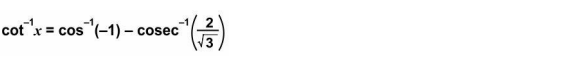OR
i) Find the domain of the function below.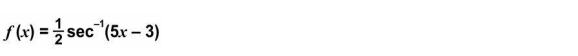ii) Find the range (principal value branch) of the function below.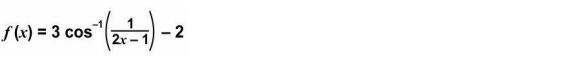Q.22.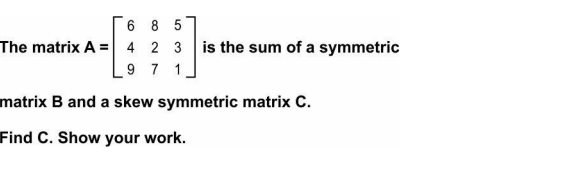Q.23 Find 𝑑𝑦/𝑑𝑥 if 𝑦 = (e^secx + 𝑥)4. Show your work.

Q.24.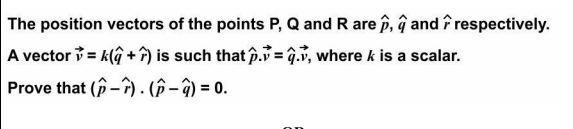OR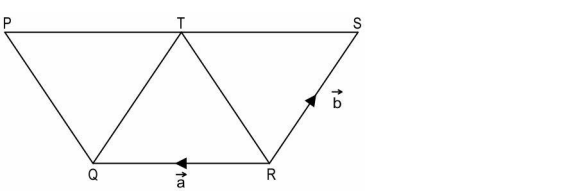In the figure below, QRST and QRTP are parallelograms.

Q.25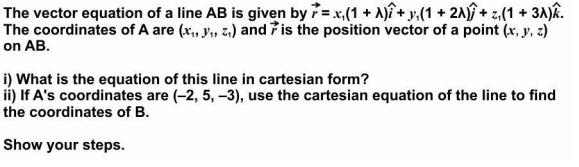## Class 12 Maths Additional  Practice Questions Section C

SECTION C
(This section comprises of short answer type questions (SA) of 3 marks each)

Q.26 Check whether the following statement is true or false.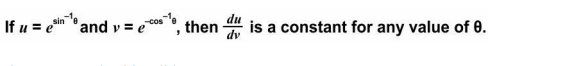Show your work with valid reason.

OR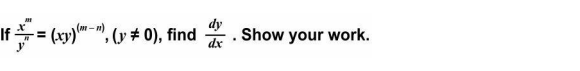Q.27 The anti-derivative of a function of the form (3x − 1)f(x), (x ≠ 1/ 3 ), is given by 3𝑥4  − (13/3)𝑥³ + (2/3)𝑥² + 𝐶, where C is the constant of integration. Find the value of f(6). Show your steps.

Q.28 Evaluate the following definite integral and show your work.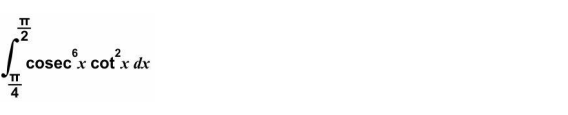Q.29. Find the particular solution when x = y = 0 for the following differential equation.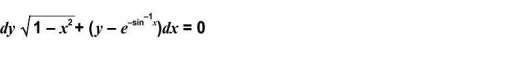OR
Find the general solution of the following differential equation.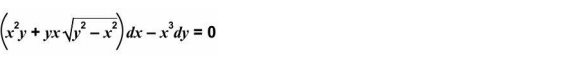Show your steps.

Q.30 Frame the below optimisation problem as a linear programming problem and determine its feasible region graphically. Bhavani Singh, a farmer, decides to raise hens and cows to make some extra money apart from his agricultural income. He wants to raise no more than 16 animals including no more than 10 hens. On an average it will cost him Rs 25 and Rs 75 per day to raise one hen and one cow respectively. He will make an average profit of Rs 12 from each hen and Rs 40 from each cow every day. He has a budget of Rs 900 per day to raise the animals. How many of each type of animals should he raise to maximise his profit?

Q.31 Nikhil has a bag of marbles that contains exactly 8 green marbles and 6 red marbles. He takes out three marbles successively without replacing any of the marbles. What is the probability that all three marbles taken out are green in colour? Show your steps.

OR

In a recreational event at a school, there were 8 students, 6 parents, and 4 teachers. To play a game, two members were selected randomly one after the other. Find the probability distribution of the number of students. Show your steps.

## CBSE Class 12 Maths Additional  Practice Questions 2023 Section D

SECTION D
(This section comprises of long answer-type questions (LA) of 5 marks each)

Q.32 Sravan is a nutritionist. He wants to create a mixture of orange juice, beetroot juice and kiwi juice that can provide 1860 mg of vitamin C, 22 mg of iron and 760 mg of calcium. The quantity of each nutrient per litre of juice is shown below.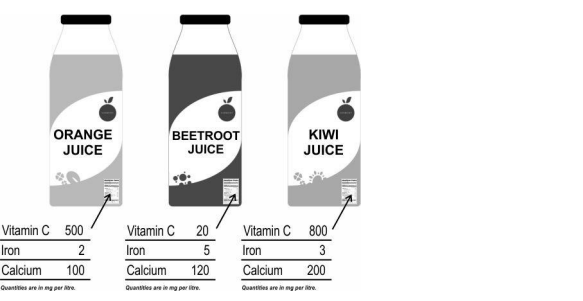Using the matrix method, find how many litres of each juice Sravan should add into the mixture. Show your work.

Q.33 Shown below is an ellipse whose equation is 𝑥²/ 81 + 𝑦²/ 36 = 1.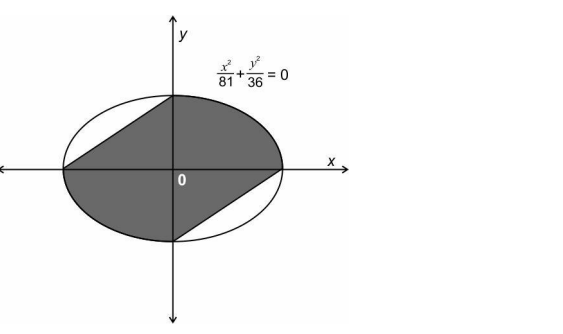Find the area of the shaded region in terms of π. Show your steps.
Q.34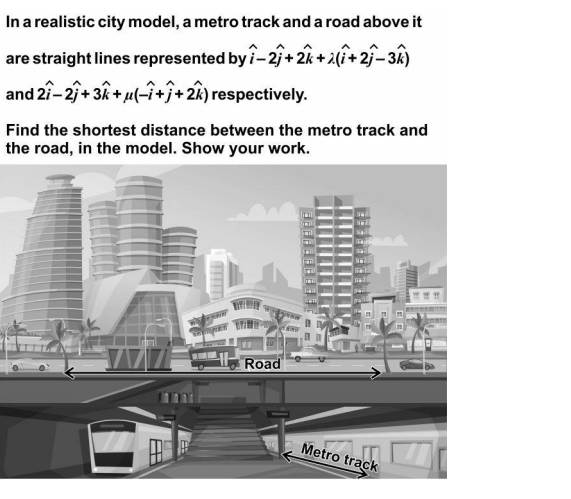OR,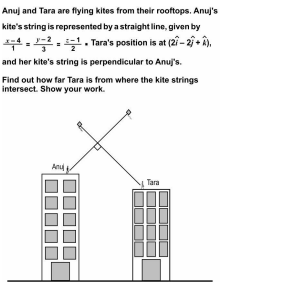Q.35. Integrate the given function and show your steps.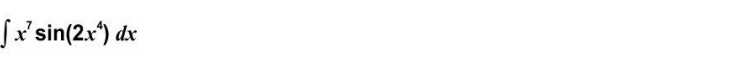OR,

Evaluate the integral and show your steps.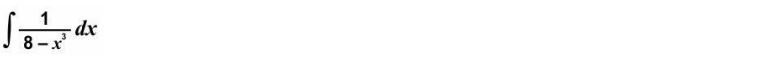## CBSE Class 12 Maths Additional  Practice Questions 2023 Section E

SECTION E
(This section comprises of 3 case-study/passage-based questions of 4 marks each with two sub-questions. First two case study questions have three sub-questions of marks 1, 1, 2 respectively. The third case study question has two sub-questions of 2 marks each.)

Q.36 Answer the questions based on the given information.
Port Blair, the capital city of Andaman and Nicobar Islands is directly connected to Chennai and Vishakapatnam via ship route. The ships sail from Chennai/Vishakapatnam to Port Blair and vice versa.
Swaraj Dweep and Shaheed Dweep are two popular tourist islands in Andaman Islands. One has to take a ferry from Port Blair to reach these islands. There are ferries that sailfrequently between the three islands – Port Blair (PB), Swaraj Dweep (SwD) and Shaheed Dweep (ShD). Shown below is a schematic representation of the ship routes and ferry routes.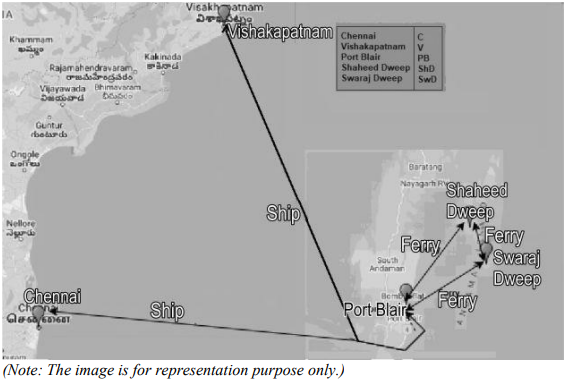X is the set of all 5 places and Y is the set of 3 places in Andaman Islands.

That is, X = {C, V, PB, SwD, ShD} and Y = {PB, SwD, ShD}.
A relation R defined on the set X is given by, R = {(𝑥1, 𝑥2): there is a direct ship or direct ferry from 𝑥1 to 𝑥2}.
A function 𝑓: Y → X is defined by, 𝑓(𝑃𝐵) = 𝑉, 𝑓(𝑆𝑤𝐷) = 𝑃𝐵, 𝑓(𝑆ℎ𝐷) = 𝑆𝑤𝐷.

i) List all the elements of R.   (1)
ii) Is the relation R symmetric? Give a valid reason.   (1)
iii) Is the relation R transitive? Give a valid reason.   (2)

OR

Check whether the function f is one-one and onto. Give valid reasons.

Q.37. Answer the questions based on the given information.
A medicinal drug administered into a human body requires some time to produce its effect on the body. The amount (in mg) of a certain medicinal drug in the bloodstream at t hours after administering the drug to an individual is given by the function:

C(t) = −t³ + 4.5t² + 54t , 0 ≤ t ≤ 10

Shown below is the graph of C(t) in the interval [0, 10]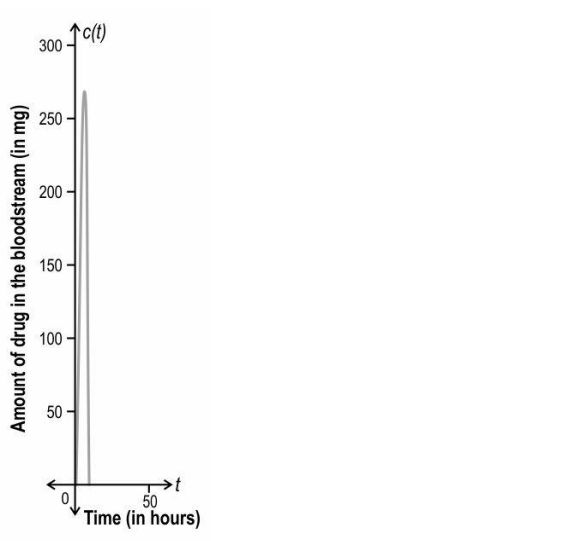i) Find the rate at which the amount of drug is changing in the bloodstream at 5 hours after administering the drug. Show your work.    (1)
ii) Show that the function C(t) is strictly increasing in the interval (3, 4).    (2)

OR

The amount of the drug in the bloodstream at which the action of the drug is maximum is denoted by Cmax.
How long after administering the drug is Cmax attained? Show your work and give valid reasons.
iii) Find the amount of drug in the bloodstream at the time when the effect of the drug is maximum. Show your work.   (1)

Q.38 Answer the questions based on the given information.

A school conducted a survey of their school staff to find their beverage preferences. Each of them picked either tea or coffee as their first preference and then with sugar or without sugar as their second preference as shown in the below tree diagram.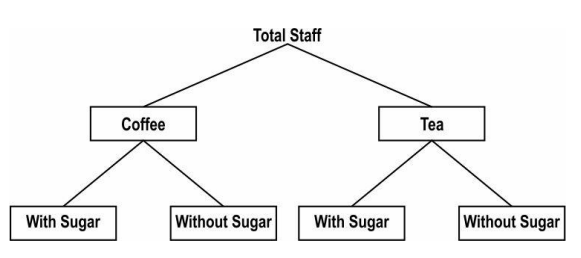Some of the insights from the survey are given below.

• 60% percent of the staff prefer coffee.
• 90% of those who prefer coffee prefer it with sugar.
• 20% of those who prefer tea prefer it without sugar.

i) What is the probability that a person selected randomly from the staff prefers a beverage with sugar? Show your steps.   (2)
ii) What is the probability that a person from the staff selected at random prefers coffee given that it is without sugar? Show your steps.     (2)

Candidates of class 12 may download the CBSE Class 12 Maths Additional Practice Question Paper 2023 and enhance their preparation for the upcoming CBSE Class 12 Board Exam 2023 by using the sample question paper provided here in pdf format.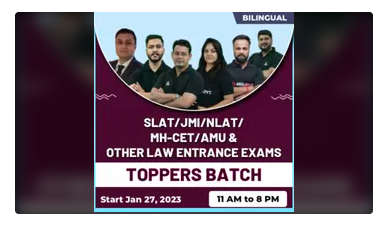## CBSE Class 12 Maths Practice Questions Previous Year

Section A: This section has very short type questions of 2 marks each.

Section B: This section has short-type questions of 3 marks each.

Section C: This section has long-type questions of 4 marks each.

## CBSE Class 12th Maths Term 2 Additional Practice Questions

1. Study the equation given belowOR

Integrate:2. Shown below is a differential equation.Find the order and the degree of the given differential equation. Give reasons to support your answer.

3. Shown below are two vectors in their component forms.For what values of p and q are the vectors collinear? Show your steps.

4. A(-1, 3, 2), B(-2, 3, -1), C(-5, -4, p) and D(-2, -4, 3) are four points in space. Lines AB and CD are parallel. Find the value of p. Show your work and give valid reasons.

5. Bhavani is going to play a game of chess against one of four opponents in an intercollege sports competition. Each opponent is equally likely to be paired against her. The table below shows the chances of Bhavani losing, when paired against each opponent.If the probability that Bhavani loses the game that day is 1/2, find the probability for Bhavani to be losing the game when paired against Opponent 3. Show your steps.

6. In the game of archery, the probability of Likith and Harish hitting the target are 2/3 and 3/4 respectively. If both of them shoot an arrow, find the probability that the target is NOT hit by either of them. Show your steps.

SECTION B

7. The first derivative of a function f(x) is given below.If f(1) = e + 3, find f(x). Show your steps.

8. Find the general solution of the differential equation given below. Show your steps.OR
Shown below is a differential equation where the value of y is 0 when x = 3.Find the value of y when x = 5. Show your steps.

9. Three vertices – A, B and D of a parallelogram ABCD are given by, A(0, -3, 3), B(-5, m – 3, 0) and D(1, -3, 4). The area of the parallelogram ABCD is 6 sq units. Using the vector method, find the value(s) of m. Show your steps.

OR10. The vector form of equations of two lines, l1 and l2 are:Show that l1 and l2 are skew lines.

SECTION C

11. Evaluate:12. Shown below is a parabola(Note: Take √2 as 1.4 and √3 as 1.7.)
OR
Shown below is the graph of f(x) = 2×2 in the first quadrant.(Note: You need not evaluate the square roots.)

13. Shown below are equations of two planes.
Plane 1: −6? − 3? − 2? = 12
Plane 2: ?⃗(2?̂ − 11?̂ − 10?̂) − 6 = 0
Is the point (-2, -3, -1) closer to plane 1 or plane 2? Show your work.

14. Read the information given below and answer the questions that follow. For an audition for a reality singing competition, interested candidates were asked to apply under one of the two musical genres – folk or classical and under one of the two age categories – below 18 or 18 and above. The following information is known about the 2000 applications received:
♦ 960 of the total applications were for the folk genre.
♦ 192 of the folk applications were for the below 18 category.
♦ 104 of the classical applications were for the 18 and above category.
a) What is the probability that an application selected at random is for the 18 and above category provided it is under the classical genre? Show your work.
b) An application selected at random is found to be under the below 18 category. Find the probability that it is under the folk genre. Show your work.

Sharing is caring!

## FAQs

### What is the CBSE Class 12 Exam Date 2022?

The Central Board of Secondary Education ios going to conduct the CBSE Class 12 Board Exams from February 15, 2023, onwards.

### Where I can get CBSE Class 12 Mathematics Practice Questions 2022?

We have given CBSE Class 12 Mathematics Practice Questions 2022 on this page. For other subjects, the students can visit the official website of Adda247 School.

### How Can I get the CBSE Class 12 Maths Additional Practice Question Paper 2023 Pdf?

CBSE Class 12 Maths Additional Practice Question Paper 2023pdf is now available on the on cbseacademic.nic.in.Although to make things easier for students here we provide the direct Link to download the CBSE Class 12 Maths Additional Practice Question Paper 2023 Pdf.

### TOPICS:

•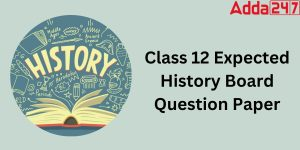Class 12 History Board Paper 2023 {Most ...
•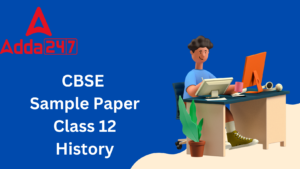Class 12 History Sample Paper 2022-23 wi...
•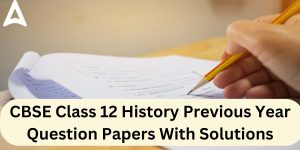CBSE Class 12 History Previous Year Ques...
•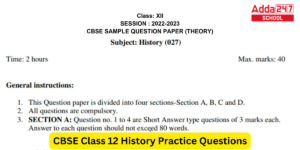CBSE Class 12 History Additional Practic...
•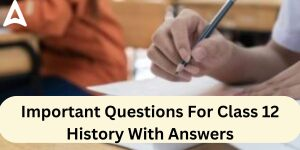Important Questions For Class 12 History...
•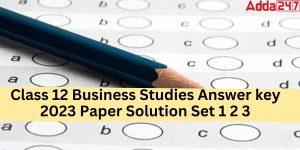Class 12 Business Studies Answer key 202...